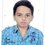# NMTC 2000

I was doing this problem

Let $\large\ f(x)=\frac { { 16 }^{ x } }{ { 16 }^{ x }+4 }$

Evalute the sum :

$\large\ f\left( \frac { 1 }{ 2000 } \right) +f\left( \frac { 2 }{ 2000 } \right) +...+f\left( \frac { 1999 }{ 2000 } \right)$

I replaced $x$ by $1-x$ and got

$\large\ f(1-x)=\frac { { 16 }^{ 1-x } }{ { 16 }^{ 1-x }+4 } =\frac { { 16 }/{ 16 }^{ x } }{ (16+4.{ 16 }^{ x })/{ 16 }^{ x } } =\frac { 16 }{ 16+4.{ 16 }^{ x } } =\frac { 4 }{ { 16 }^{ x }+4 }$

I observed that

$\large\ f(x)+f(1-x)=\frac { { 16 }^{ x } }{ { 16 }^{ x }+4 } +\frac { 4 }{ { 16 }^{ x }+4 } =1$.

Thus, i wrote the given summation of functions as :

$\large\ \left[ f\left( \frac { 1 }{ 2000 } \right) +f\left( \frac { 1999 }{ 2000 } \right) \right] +\left[ f\left( \frac { 2 }{ 2000 } \right) +f\left( \frac { 1998 }{ 2000 } \right) \right] +...+\left[ f\left( \frac { 999 }{ 2000 } \right) +f\left( \frac { 1001 }{ 2000 } \right) \right] +f\left( \frac { 1000 }{ 2000 } \right)$

$\large\ =\quad 999\quad \times \quad 1\quad +\quad f\left( \frac { 1 }{ 2 } \right)$

$\large\ =\quad 999\quad +\quad \frac { 1 }{ 2 }$

$\large\ =\quad 999\frac { 1 }{ 2 }$.

Where is wrong?

If you have more logical , easier solution, do post that.Note by Priyanshu Mishra
5 years, 10 months ago

This discussion board is a place to discuss our Daily Challenges and the math and science related to those challenges. Explanations are more than just a solution — they should explain the steps and thinking strategies that you used to obtain the solution. Comments should further the discussion of math and science.

When posting on Brilliant:

• Use the emojis to react to an explanation, whether you're congratulating a job well done , or just really confused .
• Ask specific questions about the challenge or the steps in somebody's explanation. Well-posed questions can add a lot to the discussion, but posting "I don't understand!" doesn't help anyone.
• Try to contribute something new to the discussion, whether it is an extension, generalization or other idea related to the challenge.

MarkdownAppears as
*italics* or _italics_ italics
**bold** or __bold__ bold
- bulleted- list
• bulleted
• list
1. numbered2. list
1. numbered
2. list
Note: you must add a full line of space before and after lists for them to show up correctly
paragraph 1paragraph 2

paragraph 1

paragraph 2

[example link](https://brilliant.org)example link
> This is a quote
This is a quote
    # I indented these lines
# 4 spaces, and now they show
# up as a code block.

print "hello world"
# I indented these lines
# 4 spaces, and now they show
# up as a code block.

print "hello world"
MathAppears as
Remember to wrap math in $$ ... $$ or $ ... $ to ensure proper formatting.
2 \times 3 $2 \times 3$
2^{34} $2^{34}$
a_{i-1} $a_{i-1}$
\frac{2}{3} $\frac{2}{3}$
\sqrt{2} $\sqrt{2}$
\sum_{i=1}^3 $\sum_{i=1}^3$
\sin \theta $\sin \theta$
\boxed{123} $\boxed{123}$

Sort by:

- 5 years, 9 months ago

its the easiest way and i am pretty sure that its correct

- 5 years, 9 months ago

Yes it is...correct!!

- 5 years, 9 months ago